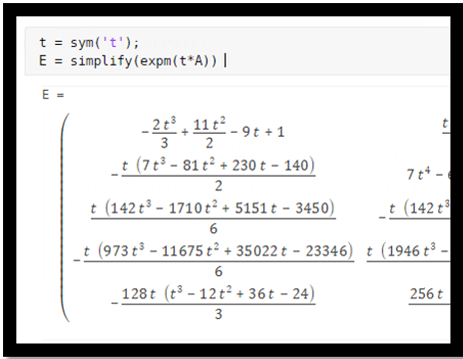# Symbolic Matrix Computation

This example shows how to perform simple matrix computations using Symbolic Math Toolbox™.Generate a possibly familiar test matrix, the 5-by-5 Hilbert matrix.

`H = sym(hilb(5)) `
```H =  $\left(\begin{array}{ccccc}1& \frac{1}{2}& \frac{1}{3}& \frac{1}{4}& \frac{1}{5}\\ \frac{1}{2}& \frac{1}{3}& \frac{1}{4}& \frac{1}{5}& \frac{1}{6}\\ \frac{1}{3}& \frac{1}{4}& \frac{1}{5}& \frac{1}{6}& \frac{1}{7}\\ \frac{1}{4}& \frac{1}{5}& \frac{1}{6}& \frac{1}{7}& \frac{1}{8}\\ \frac{1}{5}& \frac{1}{6}& \frac{1}{7}& \frac{1}{8}& \frac{1}{9}\end{array}\right)$```

The determinant is very small.

`d = det(H) `
```d =  $\frac{1}{266716800000}$```

The elements of the inverse are integers.

`X = inv(H) `
```X =  $\left(\begin{array}{ccccc}25& -300& 1050& -1400& 630\\ -300& 4800& -18900& 26880& -12600\\ 1050& -18900& 79380& -117600& 56700\\ -1400& 26880& -117600& 179200& -88200\\ 630& -12600& 56700& -88200& 44100\end{array}\right)$```

Verify that the inverse is correct.

`I = X*H`
```I =  $\left(\begin{array}{ccccc}1& 0& 0& 0& 0\\ 0& 1& 0& 0& 0\\ 0& 0& 1& 0& 0\\ 0& 0& 0& 1& 0\\ 0& 0& 0& 0& 1\end{array}\right)$```

Find the characteristic polynomial.

`syms x; p = charpoly(H,x) `
```p =  ${x}^{5}-\frac{563 {x}^{4}}{315}+\frac{735781 {x}^{3}}{2116800}-\frac{852401 {x}^{2}}{222264000}+\frac{61501 x}{53343360000}-\frac{1}{266716800000}$```

Try to factor the characteristic polynomial.

`factor(p) `
```ans =  $\left(\begin{array}{cc}\frac{1}{266716800000}& 266716800000 {x}^{5}-476703360000 {x}^{4}+92708406000 {x}^{3}-1022881200 {x}^{2}+307505 x-1\end{array}\right)$```

The result indicates that the characteristic polynomial cannot be factored over the rational numbers.

Compute the 50 digit numerical approximations to the eigenvalues.

```digits(50) e = eig(vpa(H)) ```
```e =  $\left(\begin{array}{c}1.5670506910982307955330110055207246339493152522334\\ 0.20853421861101333590500251006882005503858202260343\\ 0.01140749162341980655945145886658934504234843052664\\ 0.00030589804015119172687949784069272282565614514909247\\ 0.0000032879287721718629571150047605447313997367890230746\end{array}\right)$```

Create a generalized Hilbert matrix involving a free variable, $t$.

```t = sym('t'); [I,J] = meshgrid(1:5); H = 1./(I+J-t)```
```H =  ```

Substituting $t=1$ retrieves the original Hilbert matrix.

`subs(H,t,1) `
```ans =  $\left(\begin{array}{ccccc}1& \frac{1}{2}& \frac{1}{3}& \frac{1}{4}& \frac{1}{5}\\ \frac{1}{2}& \frac{1}{3}& \frac{1}{4}& \frac{1}{5}& \frac{1}{6}\\ \frac{1}{3}& \frac{1}{4}& \frac{1}{5}& \frac{1}{6}& \frac{1}{7}\\ \frac{1}{4}& \frac{1}{5}& \frac{1}{6}& \frac{1}{7}& \frac{1}{8}\\ \frac{1}{5}& \frac{1}{6}& \frac{1}{7}& \frac{1}{8}& \frac{1}{9}\end{array}\right)$```

The reciprocal of the determinant is a polynomial in $t$.

`d = 1/det(H) `
```d =  $-\frac{\left(t-2\right) {\left(t-3\right)}^{2} {\left(t-4\right)}^{3} {\left(t-5\right)}^{4} {\left(t-6\right)}^{5} {\left(t-7\right)}^{4} {\left(t-8\right)}^{3} {\left(t-9\right)}^{2} \left(t-10\right)}{82944}$```
`d = expand(d)`
```d =  $-\frac{{t}^{25}}{82944}+\frac{25 {t}^{24}}{13824}-\frac{5375 {t}^{23}}{41472}+\frac{40825 {t}^{22}}{6912}-\frac{15940015 {t}^{21}}{82944}+\frac{21896665 {t}^{20}}{4608}-\frac{240519875 {t}^{19}}{2592}+\frac{1268467075 {t}^{18}}{864}-\frac{1588946776255 {t}^{17}}{82944}+\frac{2885896606895 {t}^{16}}{13824}-\frac{79493630114675 {t}^{15}}{41472}+\frac{34372691161375 {t}^{14}}{2304}-\frac{8194259295156385 {t}^{13}}{82944}+\frac{7707965729450845 {t}^{12}}{13824}-\frac{55608098247105175 {t}^{11}}{20736}+\frac{37909434298793825 {t}^{10}}{3456}-\frac{197019820623693025 {t}^{9}}{5184}+\frac{10640296363350955 {t}^{8}}{96}-\frac{38821472549340925 {t}^{7}}{144}+\frac{12958201048605475 {t}^{6}}{24}-\frac{1748754621252377 {t}^{5}}{2}+1115685328012530 {t}^{4}-1078920141906600 {t}^{3}+742618453752000 {t}^{2}-323874210240000 t+67212633600000$```

The elements of the inverse are also polynomials in $t$.

`X = inv(H) `
```X =  ```

Substituting $t=1$ generates the Hilbert inverse.

`X = subs(X,t,'1') `
```X =  $\left(\begin{array}{ccccc}25& -300& 1050& -1400& 630\\ -300& 4800& -18900& 26880& -12600\\ 1050& -18900& 79380& -117600& 56700\\ -1400& 26880& -117600& 179200& -88200\\ 630& -12600& 56700& -88200& 44100\end{array}\right)$```
`X = double(X) `
```X = 5×5 25 -300 1050 -1400 630 -300 4800 -18900 26880 -12600 1050 -18900 79380 -117600 56700 -1400 26880 -117600 179200 -88200 630 -12600 56700 -88200 44100 ```

Investigate a different example.

`A = sym(gallery(5)) `
```A =  $\left(\begin{array}{ccccc}-9& 11& -21& 63& -252\\ 70& -69& 141& -421& 1684\\ -575& 575& -1149& 3451& -13801\\ 3891& -3891& 7782& -23345& 93365\\ 1024& -1024& 2048& -6144& 24572\end{array}\right)$```

This matrix is "nilpotent". It's fifth power is the zero matrix.

`A^5 `
```ans =  $\left(\begin{array}{ccccc}0& 0& 0& 0& 0\\ 0& 0& 0& 0& 0\\ 0& 0& 0& 0& 0\\ 0& 0& 0& 0& 0\\ 0& 0& 0& 0& 0\end{array}\right)$```

Because this matrix is nilpotent, its characteristic polynomial is very simple.

`p = charpoly(A,'lambda') `
`p = ${\lambda }^{5}$`

You should now be able to compute the matrix eigenvalues in your head. They are the zeros of the equation lambda^5 = 0.

Symbolic computation can find the eigenvalues exactly.

`lambda = eig(A) `
```lambda =  $\left(\begin{array}{c}0\\ 0\\ 0\\ 0\\ 0\end{array}\right)$```

Numeric computation involves roundoff error and finds the zeros of an equation that is something like lambda^5 = eps*norm(A) So the computed eigenvalues are roughly lambda = (eps*norm(A))^(1/5) Here are the eigenvalues, computed by the Symbolic Toolbox using 16 digit floating point arithmetic. It is not obvious that they should all be zero.

```digits(16) lambda = eig(vpa(A)) ```
```lambda =  $\left(\begin{array}{c}0.0005617448486395847\\ 0.0001737348850386136-0.0005342985684139864 \mathrm{i}\\ 0.0001737348850386136+0.0005342985684139864 \mathrm{i}\\ -0.000454607309358406+0.0003303865815979566 \mathrm{i}\\ -0.000454607309358406-0.0003303865815979566 \mathrm{i}\end{array}\right)$```

This matrix is also "defective". It is not similar to a diagonal matrix. Its Jordan Canonical Form is not diagonal.

`J = jordan(A) `
```J =  $\left(\begin{array}{ccccc}0& 1& 0& 0& 0\\ 0& 0& 1& 0& 0\\ 0& 0& 0& 1& 0\\ 0& 0& 0& 0& 1\\ 0& 0& 0& 0& 0\end{array}\right)$```

The matrix exponential, expm(t*A), is usually expressed in terms of scalar exponentials involving the eigenvalues, exp(lambda(i)*t). But for this matrix, the elements of expm(t*A) are all polynomials in t.

```t = sym('t'); E = simplify(expm(t*A)) ```
```E =  $\left(\begin{array}{ccccc}-\frac{2 {t}^{3}}{3}+\frac{11 {t}^{2}}{2}-9 t+1& \frac{t \left(4 {t}^{2}-27 t+33\right)}{3}& -\frac{t \left(20 {t}^{2}-117 t+126\right)}{6}& \frac{t \left(32 {t}^{2}-174 t+189\right)}{3}& -\frac{t \left(85 {t}^{2}-464 t+504\right)}{2}\\ -\frac{t \left(7 {t}^{3}-81 {t}^{2}+230 t-140\right)}{2}& 7 {t}^{4}-67 {t}^{3}+\frac{301 {t}^{2}}{2}-69 t+1& -\frac{t \left(35 {t}^{3}-293 {t}^{2}+598 t-282\right)}{2}& \frac{t \left(112 {t}^{3}-876 {t}^{2}+1799 t-842\right)}{2}& -\frac{t \left(1785 {t}^{3}-14012 {t}^{2}+28776 t-13472\right)}{8}\\ \frac{t \left(142 {t}^{3}-1710 {t}^{2}+5151 t-3450\right)}{6}& -\frac{t \left(142 {t}^{3}-1426 {t}^{2}+3438 t-1725\right)}{3}& \frac{355 {t}^{4}}{3}-\frac{3139 {t}^{3}}{3}+\frac{4585 {t}^{2}}{2}-1149 t+1& -\frac{t \left(1136 {t}^{3}-9420 {t}^{2}+20625 t-10353\right)}{3}& \frac{t \left(18105 {t}^{3}-150646 {t}^{2}+329952 t-165612\right)}{12}\\ -\frac{t \left(973 {t}^{3}-11675 {t}^{2}+35022 t-23346\right)}{6}& \frac{t \left(1946 {t}^{3}-19458 {t}^{2}+46695 t-23346\right)}{6}& -\frac{t \left(4865 {t}^{3}-42807 {t}^{2}+93390 t-46692\right)}{6}& \frac{7784 {t}^{4}}{3}-\frac{64210 {t}^{3}}{3}+\frac{93391 {t}^{2}}{2}-23345 t+1& -\frac{t \left(248115 {t}^{3}-2053748 {t}^{2}+4482036 t-2240760\right)}{24}\\ -\frac{128 t \left({t}^{3}-12 {t}^{2}+36 t-24\right)}{3}& \frac{256 t \left({t}^{3}-10 {t}^{2}+24 t-12\right)}{3}& -\frac{128 t \left(5 {t}^{3}-44 {t}^{2}+96 t-48\right)}{3}& \frac{512 t \left(4 {t}^{3}-33 {t}^{2}+72 t-36\right)}{3}& -2720 {t}^{4}+\frac{67552 {t}^{3}}{3}-49144 {t}^{2}+24572 t+1\end{array}\right)$```

By the way, the function "exp" computes element-by-element exponentials.

`X = exp(t*A) `
```X =  $\left(\begin{array}{ccccc}{\mathrm{e}}^{-9 t}& {\mathrm{e}}^{11 t}& {\mathrm{e}}^{-21 t}& {\mathrm{e}}^{63 t}& {\mathrm{e}}^{-252 t}\\ {\mathrm{e}}^{70 t}& {\mathrm{e}}^{-69 t}& {\mathrm{e}}^{141 t}& {\mathrm{e}}^{-421 t}& {\mathrm{e}}^{1684 t}\\ {\mathrm{e}}^{-575 t}& {\mathrm{e}}^{575 t}& {\mathrm{e}}^{-1149 t}& {\mathrm{e}}^{3451 t}& {\mathrm{e}}^{-13801 t}\\ {\mathrm{e}}^{3891 t}& {\mathrm{e}}^{-3891 t}& {\mathrm{e}}^{7782 t}& {\mathrm{e}}^{-23345 t}& {\mathrm{e}}^{93365 t}\\ {\mathrm{e}}^{1024 t}& {\mathrm{e}}^{-1024 t}& {\mathrm{e}}^{2048 t}& {\mathrm{e}}^{-6144 t}& {\mathrm{e}}^{24572 t}\end{array}\right)$```